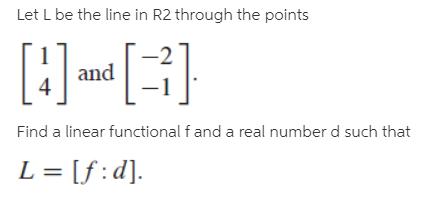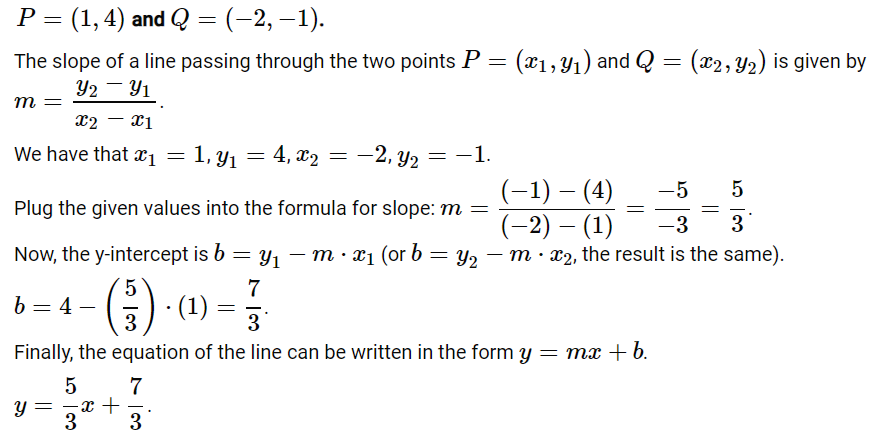# Let L be the line in R2 through the points[:] }Find a linear functional f and a real number d such thatL = [f:d].

Question
1 viewshelp_outlineImage TranscriptioncloseLet L be the line in R2 through the points [:] } Find a linear functional f and a real number d such that L = [f:d]. fullscreen
check_circle

Step 1

First, we will find the equation of a line given two points....

### Want to see the full answer?

See Solution

#### Want to see this answer and more?

Solutions are written by subject experts who are available 24/7. Questions are typically answered within 1 hour.*

See Solution
*Response times may vary by subject and question.
Tagged in